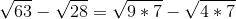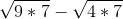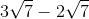# PSAT Math : How to subtract square roots

## Example Questions

### Example Question #1 : How To Subtract Square Roots

Evaluate: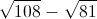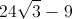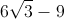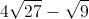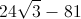Explanation:

Let us factor 108 and 81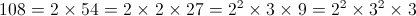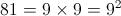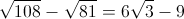### Example Question #2 : How To Subtract Square Roots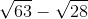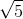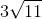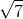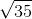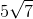Explanation:Step one: Find the greatest square factor of each radical

Forthis is, and forit is.

Therefore: